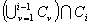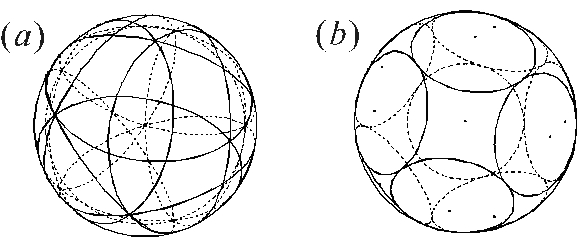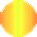PACKING AND COVERING OF CONGRUENT
SPHERICAL CAPS ON A SPHERE

TERUHISA SUGIMOTO and MASAHARU TANEMURA

 Name: Sugimoto, T. (b. Shizuoka, Japan., 1973).  Address: The Institute of Statistical Mathematics, 4-6-7 Minami-Azabu, Minato-ku, Tokyo 106-8569, Japan. E-mail: sugimoto@ism.ac.jp Fields of interest: Geometry (tiling, packing, and covering). Name: Tanemura, M. (b. Shiga, Japan., 1946).  Address: The Institute of Statistical Mathematics, 4-6-7 Minami-Azabu, Minato-ku, Tokyo 106-8569, Japan.  E-mail: tanemura@ism.ac.jp Fields of interest: Geometry, spatial statistics, mathematical crystallography.

 Abstract: Let Ci (i=1,...N) be the i-th open spherical cap of angular radius r and let Mi be its center under the condition that none of the spherical caps contains the center of another one in its interior. We consider the upper bound, rN, (not the lower bound !) of r for the case where the whole spherical surface of a unit sphere is completely covered with N congruent open spherical caps under the condition, sequentially for i=2,...,N-1, that Mi is set on the perimeter of Ci-1, and that each area of the setbecomes maximum. In this study, for N=2,...,12, we found out that the solutions of the above covering and the solutions of Tammes problem were strictly correspondent. Especially, we succeeded to obtain the exact value r10  for N = 10.

1 TAMMES PROBLEM AND OUR PROBLEM

"How must N congruent non-overlapping spherical caps be packed on the surface of a unit sphere so that the angular diameter of spherical caps will be as great as possible?" This packing problem is also called the Tammes problem and mathematically proved solutions have been known for N=1,...,12 and 24. On the other hand, the problem "How must the covering of a unit sphere be formed by N congruent spherical caps so that the angular radius of the spherical caps will be as small as possible?" is also important. It can be considered that this problem is dual to the problem of packing of Tammes. Among the problems of packing and covering on the spherical surface, the Tammes problem is the most famous. However, the systematic method of attaining these solutions has not been given.

In this study, we would like to think of the covering in connection with the packing problem. Namely, we consider the covering of the spherical caps such that none of them contains the center of another one in its interior. Such a set of centers is said to be a Minkowski set. Hereafter, we call the condition of Minkowski set of centers "Minkowski condition." If angular radii of spherical caps which cover the unit sphere under the Minkowski condition are concentrically reduced to half, the resulting spherical caps do not overlap. Then, our purpose in this study is to obtain the upper bound (not the lower bound !) of angular radius of spherical caps which cover the unit sphere under the Minkowski condition.

Suppose we have N congruent open spherical caps with angular radius r on the surface S of the unit sphere and suppose that these spherical caps cover the whole spherical surface without any gap under the Minkowski condition. Further we suppose that the spherical caps are put on S sequentially in the manner which is described just below. Let Ci be the i-th open spherical cap and let Mi be its center (i=1,...,N). Our problem is to calculate the upper bound of r for the sequential covering, such that N congruent open spherical caps cover the whole spherical surface S under the condition that Mi is set on the perimeter of Ci-1, and that each area of setbecomes maximum in sequence for i=2,...N-1. In this study, we calculate the upper bound of r for N=2,...,12 theoretically; the case N = 1 is self-evident. It is shown in this study that the solutions of our problem are strictly correspondent to those of the Tammes problem for N=2,...,12..

2 RESULTS AND CONSIDERATION

The results of our problem for N=2,...,12 are shown in Table 1. According to our method that the centers of spherical caps are chosen on the perimeters of other spherical caps under the Minkowski condition, the covering with spherical caps of angular radius r is correspondent with the packing with half-caps (the spherical cap whose angular radius is r/2 and which is concentric with that of the original cap). Let us suppose the centers of N half-caps are placed on the positions of the centers of spherical caps Ci (i=1,...,N) which are considered. At this time, we get the packing with N congruent half-caps. Then, for N=2,...,12, we find that the upper bound of angular radius of our problem with N congruent spherical caps and the value of angular diameter of Tammes problem with N congruent spherical caps are equivalent.

Accordingly, we find that the fact that the results of our problem are coincident with those of Tammes problem about N=2,...,12 at least. Especially, we obtained the exact value of r10 for N = 10, whereas Danzer has obtained the approximate range [1.154479, 1.154480] of angular diameter for N = 10. Futher, Schütte, van der Waerden, and Danzer have solved the Tammes problem for N = 7, 8, 9, 10, and 11 through the consideration on irreducible graphs obtained by connecting those points, among N points, whose spherical distance is exactly the minimal distance. Then, after establishing the theorem which states that such irreducible graphs can only have triangles and quadrangles, Schütte, van der Waerden, and Danzer proved and obtained the minimal distance r for respective values of N = 7, 8, 9 and 10. Further, they need the independent considerations for N = 7, 8, 9, 10, and 11, respectively. In contrast to this, we presented in this study a systematic method which is different from the approach by Schütte, van der Waerden, and Danzer. Namely, our method is able to obtain a solution for N by using the results for the case N-1 or N-2 successively. In addition, we have considered the packing problem from the standpoint of sequential covering. The advantages of our approach are that we only need to observe uncovered region in the process of packing and that this uncovered region decreases step by step as the packing proceeds. At least, in the cases of N£12, the solutions of Tammes problem can be found by our method. However, we may say that our method has not necessarily given a mathematical rigorous proof about our result.

Table 1: Our Upper Bound rN.Fig.  1: (a) Our sequential Covering for N = 10. (b) Our solution of Tammes problem for N = 10.

Acknowledgements

The research was partly supported by the Grant-in-Aid for Scientific Research (the Grant-in-Aid for JSPS Fellows) from the Ministry of Education, Culture, Sports, Science, and Technology (MEXT) of Japan.

References

Danzer, L., (1963) Endliche Punktmengen auf der 2-Sphäre mit möglichst großen Minimalabstand, Universität Göttingen. (Finite Point-Set on S2 with Minimum Distance as Large as Possible, Discrete Mathematics, 60, 3-66 (1986).)

Fejes Tóth, L., (1972) Lagerungen in der Ebene, auf der Kugel und im Raum, 2nd ed, Springer-Verlag, Heidelberg.

Fejes Tóth, L., (1999) Minkowski Circle Packings on the Sphere, Discrete & Computational Geometry, 22, 161-166.

Schütte, K. and van der Waerden, B. L., (1951) Auf welcher Kugel haben 5, 6, 7, 8, oder 9 Punkte mit Mindestabstand Eins Platz?, Mathematische Annalen, 123, 96-124.

Sugimoto, T. and Tanemura, M., (2001) Random Sequential Covering of a Sphere with Identical Spherical Caps, Forma, 16, 209-212.

Sugimoto, T. and Tanemura, M., (2001) Covering of a Sphere with Congruent Spherical Caps Under the Condition of Minkowski Set, Research Memorandum, ISM, 817.

Sugimoto, T. and Tanemura, M., (2002) Sphere Covering under the Minkowski Condition, Research Memorandum, ISM, 857.

Sugimoto, T. and Tanemura, M., (2003) Packing of 10, 11, and 12 Congruent Caps on a Sphere, Research Memorandum, ISM, 877.

Sugimoto, T. and Tanemura, M., (2003) Packing and Minkowski Covering of Congruent Spherical Caps on a Sphere, Research Memorandum, ISM, 901.

Sugimoto, T. and Tanemura, M., (2004) Packing and Minkowski Covering of Congruent Spherical Caps on a Sphere, under submission.